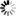Normal view

# Nonparametric statistics with applications to science and engineering / Paul H. Kvam, Brani Vidakovic

Material type:TextPublisher: Hoboken, N.J Wiley-Interscience cop. 2007Description: xiv, 420 s. ill., diagr., tab. 25 cmISBN: 9780470081471 (inb)DDC classification: 519.5 Other classification: Thi | 62P30 | 62H17 | 62-01 | 62G | 519.5 | 62
Contents:
Introduction -- Probability basics -- Statistics basics -- Bayesian statistics -- Order statistics -- Goodness of fit -- Rank tests -- Designed experiments -- Categorical data -- Estimating distribution functions -- Density estimation -- Beyond linear regression -- Curve fitting techniques -- Wavelets -- Bootstrap -- Em algorithm -- Statistical learning -- Nonparametric bayes
Tags from this library: No tags from this library for this title.Average rating: 0.0 (0 votes)
Item type Current location Call number Status Date due Barcode
Book (loan)
519.5 (Browse shelf) Available 85001942689
##### Browsing Gräsvik shelves, Shelving location: Close shelf browser
 519.5 Sannolikhetsteori och statistikteori med tillämpningar / 519.5 Basic statistics for social research / 519.5 Statistisk verktygslåda 1 : samhällsvetenskaplig orsaksanalys med kvantitativa metoder / 519.5 Nonparametric statistics with applications to science and engineering / 519.5 Matematisk statistik med tillämpningar / 519.5 Matematisk statistik med tillämpningar / 519.5 Introduction to structural equation modelling using SPSS and AMOS /

Introduction -- Probability basics -- Statistics basics -- Bayesian statistics -- Order statistics -- Goodness of fit -- Rank tests -- Designed experiments -- Categorical data -- Estimating distribution functions -- Density estimation -- Beyond linear regression -- Curve fitting techniques -- Wavelets -- Bootstrap -- Em algorithm -- Statistical learning -- Nonparametric bayes

There are no comments on this title.